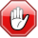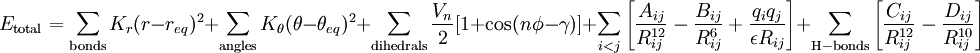# Difference between revisions of "AMBER forcefield"This article is a 'stub' page, it has no, or next to no, content. It is here at the moment to help form part of the structure of SklogWiki. If you add sufficient material to this article then please remove the {{Stub-general}} template from this page.This page contains numerical values and/or equations. If you intend to use ANY of the numbers or equations found in SklogWiki in any way, you MUST take them from the original published article or book, and cite the relevant source accordingly.

## Force field$E_{\rm total} = \sum_{\rm bonds} K_r (r - r_{eq})^2 + \sum_{\rm angles} K_\theta (\theta - \theta_{eq})^2 + \sum_{\rm dihedrals} {V_n \over 2} [1 + {\rm cos}(n\phi - \gamma)] + \sum_{i

## Parameters

#### ff02

The ff02 force field is a polarisable variant of ff99. The charges were determined at the B3LYP/cc- pVTZ//HF/6-31g* level, and hence are more like "gas-phase" charges.